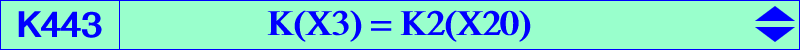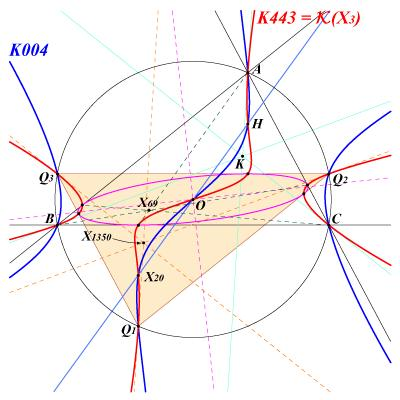X(3), X(4), X(20) infinite points of the Orthocubic K006 antipodes of A, B, C on the circumcircle vertices of the cevian triangle of X(69) and their reflections in O foci of the inconic with center O, perspector X(69) X4-OAP points, see also Table 53.See Cubics Related to Coaxial Circles §10.6. K443 is also psK(X3, X69, X3) in Pseudo-Pivotal Cubics and Poristic Triangles and spK(X4, X3) in CL055. K443 is the isogonal transform of K376. The Darboux cubic K004 and the decomposed cubic which is the union of the circumcircle and the Euler line generate a pencil of central cubics with center O passing through H, X(20) and the reflections A', B', C' of A, B, C about O. Each cubic has the same asymptotic directions as one of the isogonal pKs of the Euler pencil. This pencil also contains (apart K004) the cubics K047, K080, K426, K443, K566 corresponding to K002, K003, pK(X6, X3146), K006, K005 respectively. See also Table 54 where K443 is mentioned in one line and two columns showing that it belongs to three pencils of cubics generated by : • K003 and K187, line Q = X3, • K002 and K028, column P = aQ, • K004 and the union of the Euler line with the circumcircle, column P = [X20], already mentioned above. At last, K443 is also a member of the pencil generated by K006 and the union of the Jerabek hyperbola with the line at infinity. *** Locus property Let Pa be the orthopole of the perpendicular at P to the line AP. Define Pb and Pc cyclically. The triangles ABC and PaPbPc are perspective if and only if P lies on K443, together with le line at infinity and the sidelines of ABC. (Problem raised by Ercole Suppa, Euclid 5737, 2023-02-23).